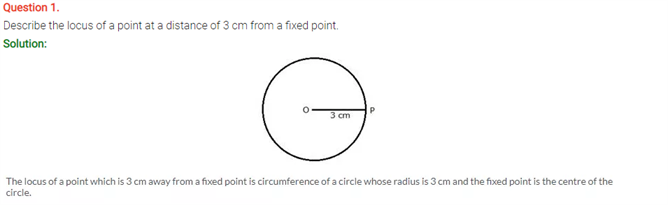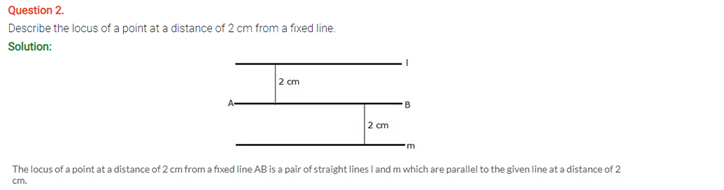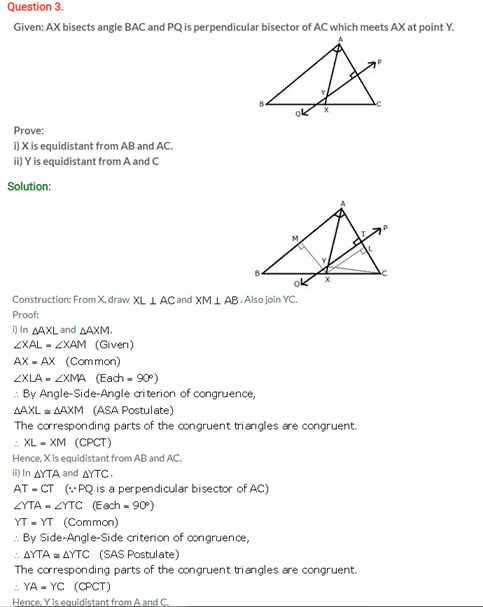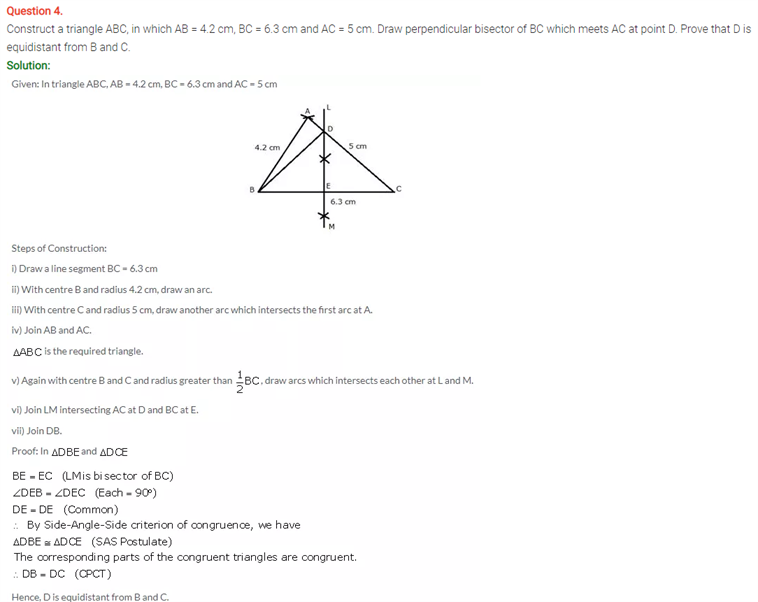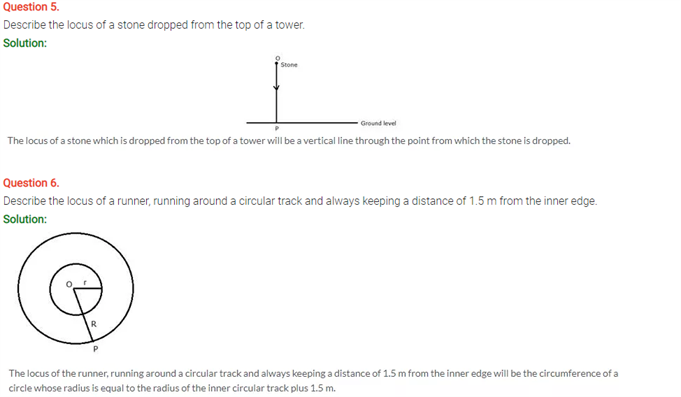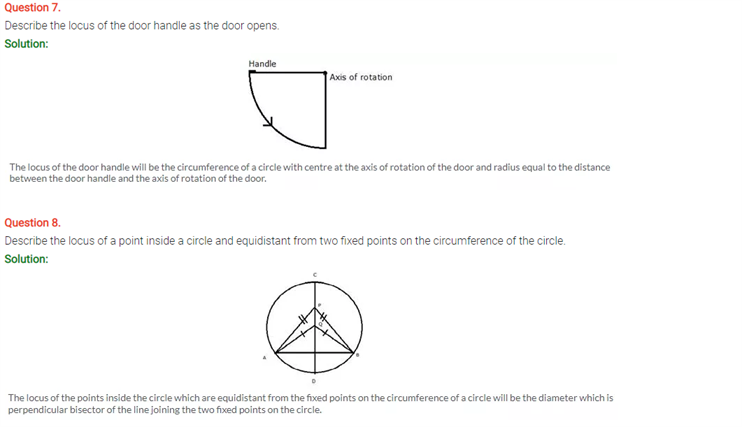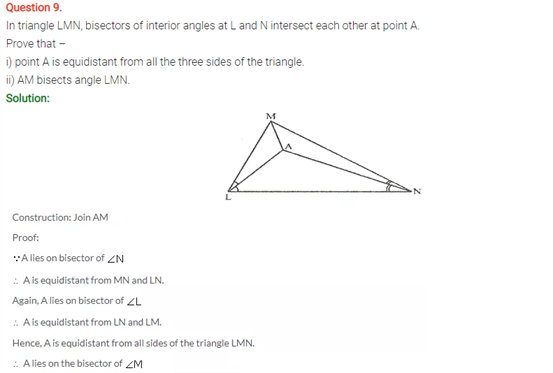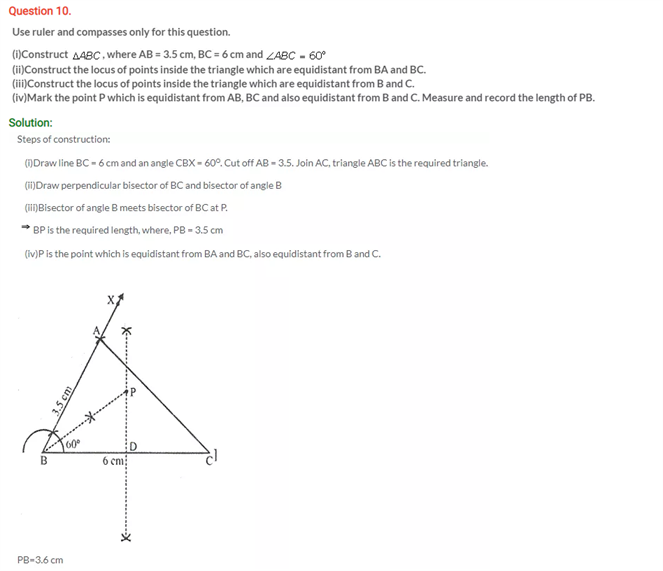# Selina Concise Maths Solution for ICSE Class 10 Chapter 16 Loci (Locus and Its Constructions)

## Selina Class 10 Maths Solutions for Chapter 16

If we consider that every infinite point packed together constitutes a plane, then if certain points meet certain rules then they are said to form a certain shape, a line or a curve. Solving the solutions on locus and its application from the ICSE Class 10 Maths Selina Solutions will ensure a better grasp of this chapter. From a geometrical point of view, we tend to use the word locus to mean the shape formed by a certain set of points. We will find a mathematical meaning of the terms straight line and ellipse. These questions below belong to the above concept.

Students can view the Class 10 Selina Maths ICSE Solutions Chapter 16 Loci (Locus and Its Construction) below: